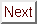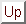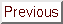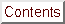Next: References Up: No Title Previous: Effect of Tilt

# Estimation of the Dimensionless ``Cleanliness'' Parameter

Cleanliness Parameter Lambdatr

In Section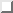, the dimensionless cleanliness parameter Lambdatr is discussed. Lambdatr >> 1 indicates dirty-limit superconducting behavior typical of disordered materials, while Lambdatr << 1 is indicative of clean-limit superconducting behavior typical of single-crystals. Below Lambdatr is estimated for C4KHg.

The standard definition of Lambdatr iswhere l is the mean-free path, andis the BCS coherence length. Since for C4KHg the only experimental data related to vF is for in-plane transport, only the in-plane Lambdatr can be calculated.

The Fermi velocity can be estimated for C4KHg by using the Shubnikov-deHaas data of Timp et al. in conjunction with the rigid-band model for the graphitic pi carriers. In this context, the rigid-band model implies the use of graphitic band parameters for the shape of the pi-carrier piece of the GIC's Fermi surface. In the rigid-band picture, only the size of the pi piece of the Fermi surface changes upon intercalation, thus changing kF and vF. There is ample precedent for using the rigid-band model to estimate electronic parameters of the donor GIC's,[245,79] although the approximations made are not as valid for stage 1 as for higher stages. Since c-axis transport is believed to be through the intercalant bands rather than the graphitic pi bands,[4,229] the rigid-band analysis is inapplicable. Therefore, there is no good way of estimating vF for the c-axis direction, and no reasonable method to calculate xi0 or Lambdatr.

Timp and colleagues measured a maximum in-plane Shubnikov-deHaas frequency of numax = 2490 T for C4KHg. Since kF = sqrt2e numax / hbar c , this gives kF = 2.75× 107 cm-1. For graphitic pi bands, m* = hbar2 kF / p0, where p0 = 1.08× 10-19 erg-cm. This formula gives m* = 0.31 me for the in-plane effective mass of C4KHg, in excellent agreement with the m* = 0.32 me found using reflectivity. Using vF = hbar kF / m*, the result is vF = 1.0× 108 cm/s. Then xi0 = 9000 Å.

The main obstacle in estimating la, the mean-free-path for in-plane transport, is that the in-plane resistivity has not been reported below 100 K (see Section). However, rhoc measurements have been published down to liquid-helium temperature. In order to make further progress, a reasonable procedure is to estimateThis procedure gives a rough estimate of rhoa(4.2K) 0.7 mu Omega-cm, which compares well with the measured rhoa(4.2K) = 0.6 muOmega-cm for C8KHg.

For the mean-free path, the relationship isFor an ellipsoidal Fermi surface, the carrier density n is given bywhere Ic is the c-axis repeat distance. Then l isPlugging in the numbers gives la 9100 Å at liquid helium temperature.

Finally now it is possible to calculate Lambdatr 0.86. This is indicative of fairly clean superconductivity for C4KHg for in-plane transport ( vecH || ^c), although not really the ``clean limit''.

All these formulae can be reduced to the expressionwhere rhoa is in Omega-cm, k F is in cm-1, Tc is in K, and Ic is in Å. Getting kF from the C8KHg maximum SdH frequency of 1490 T, a similar calculation for C8KHg shows Lambdatr = 0.36. For C8K, using kF = 4.7× 107 cm-1 and using rhoa = 0.08 mu Omega-cm at 4 K (this is actually the value for C8Rb), Lambdatr is 22. The ``dirty'' value of Lambdatr for C8K is a result of its lower Tc and Ic that go into the equation above.Next: References Up: No Title Previous: Effect of Tilt

alchaiken@gmail.com (Alison Chaiken)
Wed Oct 11 22:59:57 PDT 1995